# Python - trajectory of a mass in a gravitational frield

• wigglywoogly

## Homework Statement

This is just a project for fun, not homework.
I'm writing something in python that takes in an apple's initial position vector and initial velocity vector, and for a given gravitational field due to a planet, returns the next position vector after a small time increment, until the apple crashes into the planet's surface and the program halts (or the apple flies off to infinity and the user halts it.)

Eventually I'd like to use a Tkinter GUI to show the trajectory in real time but I'll cross that bridge when I come to it.

## Homework Equations

* Newton's law of gravity (vector form) (I'm sorry but I don't know latex)

* The following recursive relationships:

v(t) = v(t-1) + dv(t) = v(t-1) + a(t) * dt

p(t) = p(t-1) + dp(t) = p(t-1) + v(t) * dt

where dt is a small change in t

## The Attempt at a Solution

I won't bore you with my entire code (and the many comments to myself!), but here's the bare bones.

I define a vector field to represent the force of gravity at every point on the x-y plane (I set the planet to be centred on the point (0, 0)):

def Fgrav((x, y)):
return ( -1*G*M*m/(math.pow(math.hypot(x, y), 3)) * x -1*G*M*m/(math.pow(math.hypot(x, y), 3)) * y )

I tried a couple of other ways too, but I keep getting the same bad results (more on that later) for example

def Fgrav((x, y)):
return ( -1*G*M*m/(math.pow(math.hypot(x, y), 2)) * math.cos(math.atan2(x, y)) ,
-1*G*M*m/(math.pow(math.hypot(x, y), 2)) * math.sin(math.atan2(x, y)) )

Then I write a loop to find next period position, given current position and current velocity.

The looping process begins with the initial position vector, p0 (which is preloaded). The current period position variable, pt, takes the value p0 and is inputted into my vector field to return a vector value for force there (for the current period t):

Fgravt = Fgrav(pt)

Period t force is divided by m to give period t acceleration:

at = Fgravt / m

Which is used to give period t change in velocity (deltat is 1 but can be varied to adjust level of 'graininess')

deltavt = (at*deltat, at*deltat)

Current period velocity, vt, takes the initial value v0 (which is preloaded.) This is combined with deltav to return next period velocity:

vnext = (vt+deltavt, vt+deltavt)

A similar process returns next period position, given current period velocity:

deltapt = (vt*deltat, vt*deltat)

pnext = (pt+deltapt, pt+deltapt)

And all these values get stored in stacks for the last 10 periods. When we calculate the apple's position for the next period it gets stored at the top of the stack so it can be used as the basis for next period's calculation of next-next period's positon, and so on.

I get lists of position vectors at the end of this process which I can plot (I can't work out how to get excel to plot my ordered pairs so I have been reduced to using an online tool!). I include one for your examination. (Just to be clear, it begins at the point (0, 7million) and spirals out clockwise.)

The problem is that the apple seems to violate energy conservation: it always flies off to infinity. No matter how carefully I set the velocity to the critical value for circular motion at the beginning, it always spirals out like below. Even when the initial velocity is a little less than what would be required for circular motion.

I have tried more complicated things like introducing a circular set of pixels to represent the planet;s atmosphere in which the apple experiences a force of drag opposite to its velocity but that just went horribly wrong...

Sorry for the long post.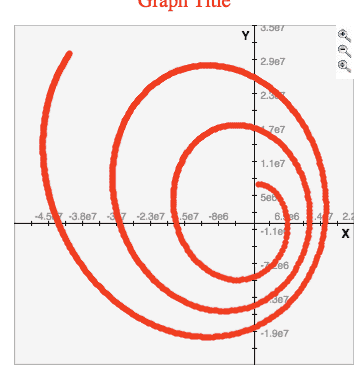Last edited:

I thought I might add some more pictures. These are from an older version of the code, calibrated differently (in all but one, the planet is not centred on (0, 0), and some have different values for G, M and deltat). But I was having essentially the same problem. Also apologies for the ad-hoc way they're labeled (or not labeled).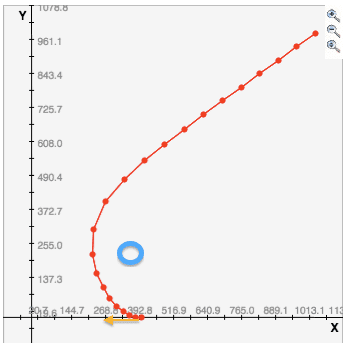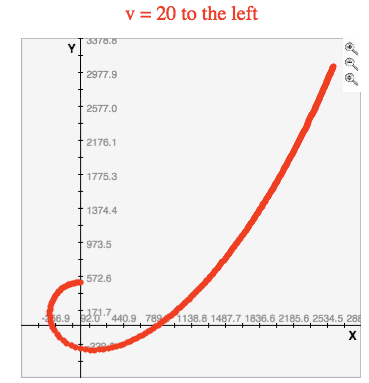this 0 initial velocity case went ok: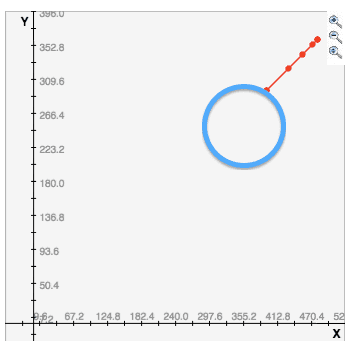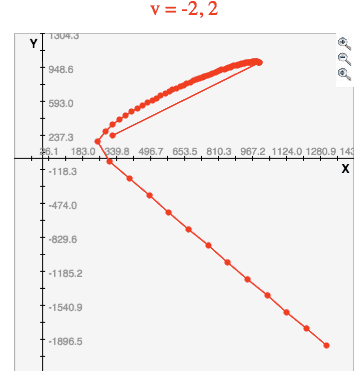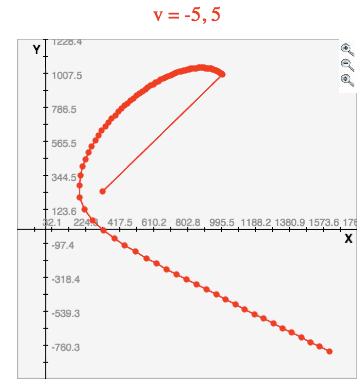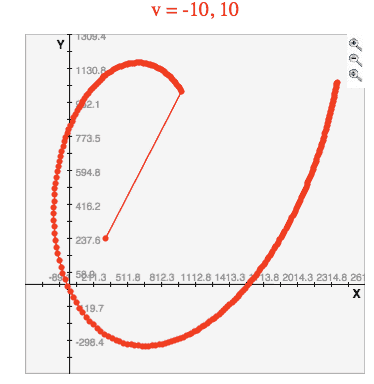Code:
return ( -1*G*M*m/(math.pow(math.hypot(x, y), 2)) * math.cos(math.atan2(x, y)) ,
-1*G*M*m/(math.pow(math.hypot(x, y), 2)) * math.sin(math.atan2(x, y)) )
... it is probably easier to find bugs if you build up simpler expressions before returning the calculated values.

IOW, use temporary variables to store the computed value for the hypotenuse and sine or cosine, and then use those variables when you calculate the hor. and vertical components of the gravitational force.

You should verify that your formulas are giving you the correct values by doing a couple of the calculations by hand.

Code:
math.pow(math.hypot(x, y), 2)
you can do this calculation: x*x + y*y. I never use any pow() function if all I'm doing is squaring a number. Although you probably wouldn't notice a difference, but multiplication is many times faster than a call to a library function such as pow().

And are you sure you want arctan(x/y) and not arctan (y/x)?

Thanks for the advice, I have changed the vector field to use fewer calls to math library. The function seems to work fine, it returns exactly what we expect. Also, you're right about arctan, was a typo.

However the problem is still the gradual increase in tangential velocity that causes the apple to deviate from circular motion. Could this be due to computer rounding errors? Please see the most recent attached picture.

Note that in the apple begins near the centre and spirals outwards, clockwise. It began with the critical velocity for circular motion, accurate to 6 decimal places.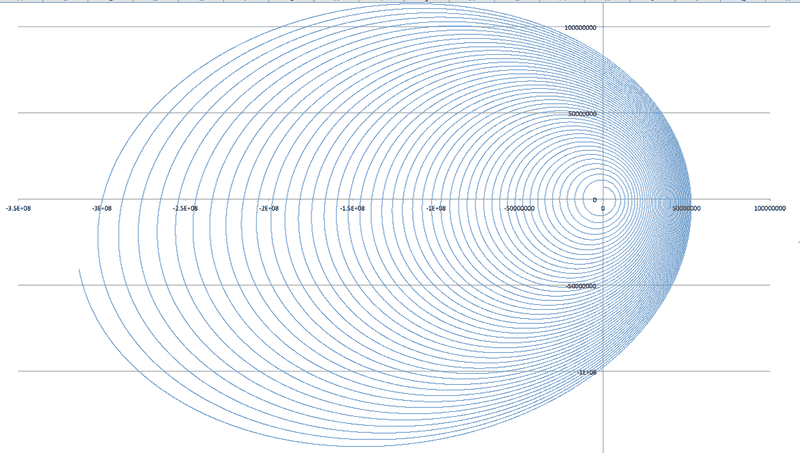Note that in the apple begins near the centre and spirals outwards, clockwise. It began with the critical velocity for circular motion, accurate to 6 decimal places.
Six decimal places might not be enough precision.